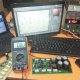Breaking News
Home / how to make inverter / how to make inverter generator

# how to make inverter generator

## how to make inverter generator:

For making an inverter is very easy. What is an inverter? An inverter is a way to convert the voltage. It can be low to high or high to low voltage. normally we make low voltage to high voltage according to our needs. But we have to know that power can change the position only. It can’t increase. Let’s go to discuss. Normally we use 12 voltage by making 220 or 110 voltage inverter. If we use 12 voltage 100 amperes Battery. we know that Voltage X Ampere= Watts. so our voltage is 12 and amperes is 100. then 12 X 100= 1200 Watts. Our Battery is 1200 Watts. Let’s go to invert the watts to AC voltage. If we need 220 voltage in AC. we know that Watts/Voltage= ampere. So, our Battery Watts is 1200 and we need voltage is 220. 1200/220=5.454545. So if we use the 220 Voltage AC in 5.45 amperes. our inverter will run for 1 hour. or if we use 220 AC voltage in 2 amperes then? 220X2=440, 1200/440=2.72. so In AC 220 voltage 2 ampere we can use the battery 2.72 hours. So we can use the inverter according to our Battery Watts. we know the inverter power is the Battery Watts. the Watts just convert the voltage and amperes.how to make inverter generator

It’s a simple diagram circuit for making knowledge for learning people.

Here have a Video for you, how to make inverter generator

If you like our post please share and subscribe our channel. like our facebook page Electronicshelpcare

transistor circuit diagram of 2sa1943 and 2sc5200

Thanks a lot to be with us. If you want another post then please visit our website.

We have another post for you. like as repairing amplifier,

Please like comment and share our post.